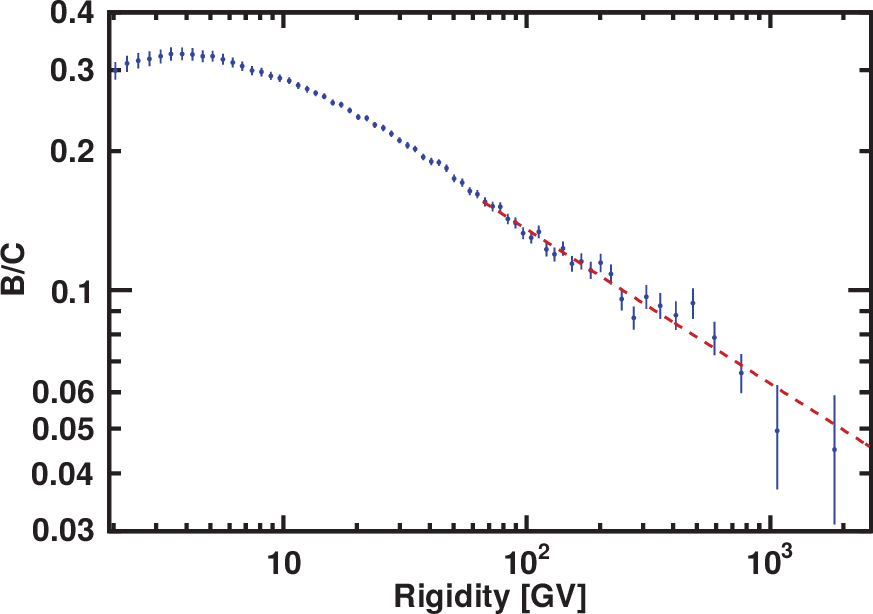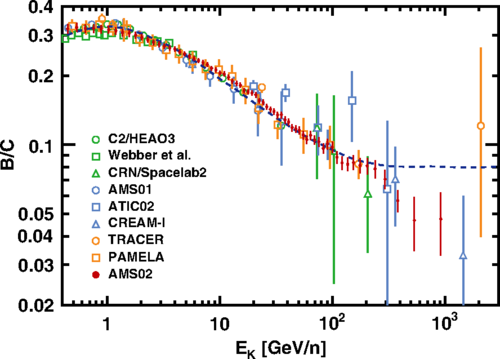# Precision Measurement of the Boron to Carbon Flux Ratio

Carbon nuclei in cosmic rays are thought to be mainly produced and accelerated in astrophysical sources, while boron nuclei are entirely produced by the collision of heavier nuclei, such as carbon and oxygen, with the interstellar matter. Therefore, the boron to carbon flux ratio (B/C) directly measures the average amount of interstellar material traversed by cosmic rays. In cosmic ray propagation models, where cosmic rays are described as a relativistic gas scattering on a magnetized plasma, the B/C ratio is used to constrain the spatial diffusion coefficient $D$, as the B/C ratio is proportional to $1/D$ at high rigidities $R$. The diffusion coefficient dependence on rigidity is $D \propto R^{-\delta}$, where $\delta$ is predicted to be $\delta = −1/3$ with the Kolmogorov theory of interstellar turbulence [A. N. Kolmogorov, Dokl. Akad. Nauk SSSR 30, 301(1941)], or $\delta = −1/2$ using the Kraichnan theory [R. H. Kraichnan, Phys. Fluids 8, 1385 (1965)]. The measured B/C spectral index $\Delta$, obtained from a fit at high rigidities of the $({\rm B}/{\rm C})=kR^{\Delta}$, approaches the diffusion spectral index $\delta$ asymptotically ($\Delta = \delta$) and $k$ is the normalization constant.

AMS precisely measured the B/C ratio in cosmic rays in the rigidity range from 1.9 GV to 2.6 TV. This measurement is based on 2.3 million boron nuclei and 8.3 million carbon nuclei collected by AMS during the first 5 years of operation onboard the ISS. In this measurement the total error is ∼3% at 100 GV. Figure 1 shows the measured B/C ratio.Figure 1. The AMS boron to carbon ratio (B/C) as a function of rigidity in the interval from 1.9 GV to 2.6 TV based on 2.3 million boron and 8.3 million carbon nuclei. The dashed line shows the single power law fit starting from 65 GV with index $\Delta=−0.333±0.014(\rm fit)\pm0.005(\rm syst)$.

As seen in Figure 1, the B/C ratio increases with rigidity reaching a maximum at 4 GV then decreases. The B/C ratio does not show any significant structures. Above 65 GV the B/C ratio measured by AMS is well fit with a single power law $kR^{\Delta}$ with a $\chi^2/\mathrm{d.o.f} = 14/24$ and a spectral index $\Delta=−0.333±0.014(\rm fit) \pm 0.005(\rm syst)$ in good agreement with the Kolmogorov theory of turbulence which predicts $\Delta = -1/3$ asymptotically.

Figure 2 shows the AMS B/C ratio together with recent results. Also shown in blue dash line is the prediction for the B/C ratio from an important theoretical model [R. Cowsik and T. Madziwa-Nussinov, Astrophys. J. 827, 119 (2016)], which explains the AMS positron fraction [L. Accardo et al., Phys. Rev. Lett. 113, 121101 (2014)] and antiproton results [M. Aguilar et al., Phys. Rev. Lett. 117, 091103 (2016)] by secondary production in cosmic ray propagation. The model shown is ruled out by this measurement.Figure 2. The AMS B/C ratio together with recent results and the prediction for the B/C ratio from the theoretical model [R. Cowsik and T. Madziwa-Nussinov, Astrophys. J. 827, 119 (2016)] (blue dash line), which explains the AMS positron fraction [L. Accardo et al., Phys. Rev. Lett. 113, 121101 (2014)] and antiproton results [M. Aguilar et al., Phys. Rev. Lett. 117, 091103 (2016)] by secondary production in cosmic ray propagation. The model shown is ruled out by this measurement.

In conclusion, the B/C ratio does not show any significant structures. Above 65 GV the B/C ratio can be described by a single power law of $\Delta =−0.333\pm0.014(\rm fit)±0.005(\rm syst)$, in good agreement with the Kolmogorov theory of turbulence which predicts $\Delta = -1/3$ asymptotically.